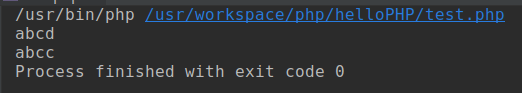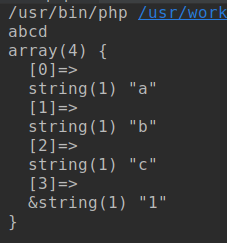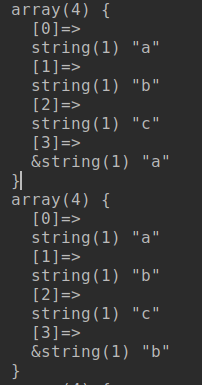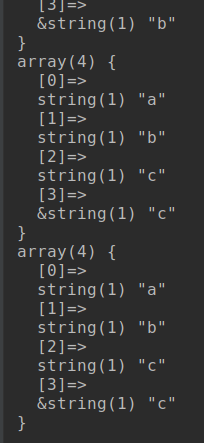# 问题

```<?php
\$arr = [
'a', 'b',
'c', 'd',
];

foreach (\$arr as &\$each){
echo \$each;
}
echo PHP_EOL;
foreach (\$arr as \$each){
echo \$each;
}
```# 问题分析

```<?php
\$arr = [
'a', 'b',
'c', 'd',
];

foreach (\$arr as &\$each){
echo \$each;
}
echo PHP_EOL;
\$each = '1';
var_dump(\$arr);
```php的foreach会在每次循环时,讲当前元素赋值给each, 然后进入循环体

• 第一次遍历, 将arr赋值给each, 相当于arr=arr, 此时arr为: ['a', 'b', 'c', 'a']

• 第二次遍历, 将arr赋值给each, 相当于arr=arr, 此时arr为: ['a', 'b', 'c', 'b']

• 第三次遍历, 将arr赋值给each, 相当于arr=arr, 此时arr为: ['a', 'b', 'c', 'c']

• 第四次遍历, 将arr赋值给each, 相当于arr=arr, 此时arr为: ['a', 'b', 'c', 'c']

```<?php
\$arr = [
'a', 'b',
'c', 'd',
];

foreach (\$arr as &\$each){
}
foreach (\$arr as \$each){
var_dump(\$arr);
}
```